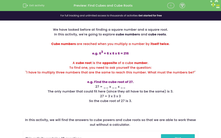# Find Cubes and Cube Roots

In this worksheet, students will find the answers to cube powers and cube roots to enable them to work these out without a calculator.Key stage:  KS 4

Year:  GCSE

GCSE Subjects:   Maths

GCSE Boards:   Pearson Edexcel, OCR, Eduqas, AQA,

Curriculum topic:   Number, Indices and Surds

Curriculum subtopic:   Structure and Calculation Powers and Roots

Popular topics:   Square Numbers worksheets

Difficulty level:#### Worksheet Overview

We have looked before at finding a square number and a square root.

In this activity, we're going to explore cube numbers and cube roots.

Cube numbers are reached when you multiply a number by itself twice.

e.g. 63 = 6 x 6 x 6 = 216

A cube root is the opposite of a cube number.

To find one, you need to ask yourself the question:

"I have to multiply three numbers that are the same to reach this number. What must the numbers be?"

e.g. Find the cube root of 27.

27 = __ x __ x __

The only number that could fit here (since they all have to be the same) is 3.

27 = 3 x 3 x 3

So the cube root of 27 is 3.

In this activity, we will find the answers to cube powers and cube roots so that we are able to work these out without a calculator.

### What is EdPlace?

We're your National Curriculum aligned online education content provider helping each child succeed in English, maths and science from year 1 to GCSE. With an EdPlace account you’ll be able to track and measure progress, helping each child achieve their best. We build confidence and attainment by personalising each child’s learning at a level that suits them.

Get started